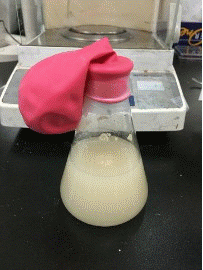# Fermentation Batch Reactor

For most of what we experience in everyday life, it is rare that one can directly link the obvious outcomes with their underlying theoretical grounds. Equations and plots seem such a long distance toward their practical applications. I regard this project as an important one which links observations of a simple experiment to the complex differential equations in reaction mechanics. This mini-project comes from a homework in reaction engineering, a course I had enrolled in during college. The experiment is simple that any person can carry out using easily accessible materials. The main objective is to construct a batch reactor that can exhibit fermentation with yeast, then quantify the reactions using what we have learned on class. (~age 21, 2017)

Two commercially available sugar-sweetened beverages, glucose solution, and water are used to explored how the sugar content in them affects the fermentation rate of rapid yeast. The anaerobic fermentation of yeast in anaerobic environment is:

C6H12O6 (monosaccharide) → 2C2H5OH (ethanol) + 2CO2 (carbon dioxide) + 2ATP

In this experiment, glass containers are filled with the solutions, then instant yeast is added the each container for production of carbon dioxide. A balloon is used for trapping the gases and is used as a volume sensor, where its dimensions are measured for calculating the volume of generated CO2. The molar concentration of CO2 is calculated using the ideal gas equation PV = nRT, and the ethanol production rate is calculated by relating with the proposed reaction and using finite different method.

Figure 1. Snapshots of balloon-sealed containers with added yeast at different times.

Figure 2. Volume of balloon (Vballoon) vs time (min).

Assume an inner air pressure of P = 1atm, a temperature of T = 310K (37°C). From the ideal gas equation, the relationship between the number of moles of CO2 and its volume is n = 3.931×10-5V, which according to the reaction, also equals the number of moles of ethanol. The molar concentration of ethanol is calculated by dividing the number of moles by its volume. And by using finite difference method of the first derivative, the rate of increase for molar concentration of ethanol (rC2H5OH) is calculated (Fig. 3).

Figure 3. Increase rate of molar concentration of ethanol (rC2H5OH) vs time (min).

It can be seen that in addition to pure water (Negative), the other three sugar-containing solutions have a maximum formation rate at the beginning (marked by the blue arrow). Wherein the ethanol production rate of glucose solution is eventually lower than 0(mM/min), it is presumed either this is caused by measurement errors or that carbon dioxide is dissolved back into the liquid, causing a decrease in volume, not a decrease in the amount of ethanol.

Here the production rate of ethanol in glucose solution started at a very high value (8.29mM/min), followed by fruit tea (4.17mM/min), and then raspberry juice (3.36mM/min). However, the sugar concentration of raspberry juice is higher than that of fruit tea. There are two factors that may be affected: the type of sugar and the pH value. Among them, the pH of fruit tea is between 5.0 and 6.0 and the pH of raspberry juice is between 2.3 and 2.52. However, the optimal living environment pH of yeast is 4.5 to 5.0, so it is speculated that the acidic environment of raspberry juice inhibits the activity of yeast and reduces rC2H5OH. In addition, only glucose exists in the glucose solution, but there is sucrose in both raspberry juice and fruit tea. Sucrose can be broken down by the yeast and producing ethanol twice as much as the same concentration of glucose. This explains why the final balloon volume (408.69cm3) of fruit tea is greater than the final balloon volume of the glucose solution (361.03cm3).

As being a simple hands-on experiment, this project successfully delivered the knowledge and allowed me to learn the fundamentals through practice, by which creating a connection between reality and theory.